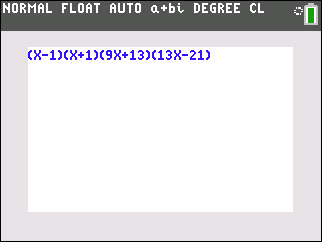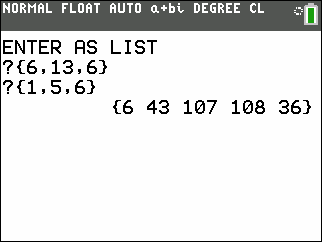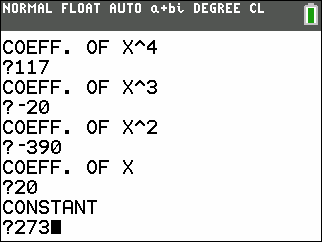Basics Archives Community Services ProgrammingHome :: Archives :: File Archives :: POLYOPSPOLYOPS

FILE INFORMATION

polyops.zip
 Filename polyops.zip (Download) Title POLYOPS Description This program multiplies, divides, and factors polynomials. When you make a choice of either one of the alternatives, you are asked to enter the coefficients of the polynomial/s. If you choose to multiply or divide, you enter the coefficients of the polynomials as lists, and you will get the coefficients of the product resp. the quotient and the remainder. If you want to factor, ( the program finds all of the linear factors of a polynomial ) you first give the degree, and then the coefficients of the p. The program then factors the polynomial. If there are irrational or imaginary/complex roots in addition to rational ones, the program gives you the coefficients of the remaining polynomial. If you want to multiply more than two polynomials, the product is in L3. Press ENTER and choose multiply again, and press L3 by the first question mark and insert the coefficients of the third polynomial by the second question mark. Similarly if you want to divide with more than one polynomial, the quotient is in L4. Author Anders Tiberg (anders.tiberg@telia.com) Category TI-84 Plus C Silver Edition/CE BASIC Math Programs File Size 1,979 bytes File Date and Time Sat Nov 12 09:24:54 2016 Documentation Included? Yes

SCREEN SHOTSREVIEWS

There are no reviews for this file.

LEAVE FEEDBACK

Questions, comments, and problems regarding the file itself should be sent directly to the author(s) listed above.

Report inappropriate or miscategorized file (requires an account; or you may email us directly)